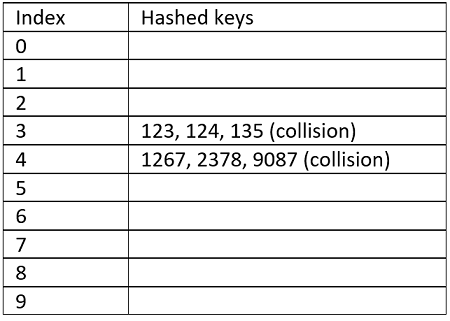# Collisions in Hashing and Collision Resolution Techniques

In this article, we are going to learn what collision is and what popular collision resolutions are?
Submitted by Radib Kar, on July 01, 2020

Prerequisite: Hashing data structure

## Collisions

Hash functions are there to map different keys to unique locations (index in the hash table), and any hash function which is able to do so is known as the perfect hash function. Since the size of the hash table is very less comparatively to the range of keys, the perfect hash function is practically impossible. What happens is, more than one keys map to the same location and this is known as a collision. A good hash function should have less number of collisions.

To understand what collision is let's check the below example,

```Say, the set of keys are;
{123, 124, 135, 1267, 2378, 9087}
and hash table size is 10(0-9 indices)

Now,
If our hashing function is
F(x)=digits in x

Then
123->3
124->3
135->3
1267->4
2378->4
9087->4
```

The hash table will look like,In the above example, we can see though there are 10 indices only 2 are being used and the collision rate is too high. 123, 124, 135 have collided while 1267, 2378, 9087 have collided.

```#include <bits/stdc++.h>
using namespace std;

//collision
int main()
{

//set of input numbers
vector<int> arr{ 123, 124, 135, 1267, 2378, 9087 };

//using hashh function f(x)=no of digits in x
cout << "using hashh function 1\n";
for (int a : arr) {
cout << a << "->" << to_string(a).length() << endl;
}

return 0;
}
```

Output:

```using hashh function 1
123->3
124->3
135->3
1267->4
2378->4
9087->4
```

## Collision Resolution Techniques

Collision resolution is finding another location to avoid the collision. The most popular resolution techniques are,

Open addressing can be further divided into,

1. Linear Probing
3. Double hashing

Top MCQs

Languages: » C » C++ » C++ STL » Java » Data Structure » C#.Net » Android » Kotlin » SQL
Web Technologies: » PHP » Python » JavaScript » CSS » Ajax » Node.js » Web programming/HTML
Solved programs: » C » C++ » DS » Java » C#
Aptitude que. & ans.: » C » C++ » Java » DBMS
Interview que. & ans.: » C » Embedded C » Java » SEO » HR
CS Subjects: » CS Basics » O.S. » Networks » DBMS » Embedded Systems » Cloud Computing
» Machine learning » CS Organizations » Linux » DOS
More: » Articles » Puzzles » News/Updates